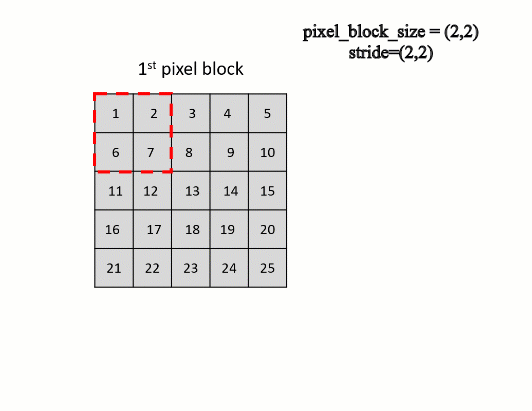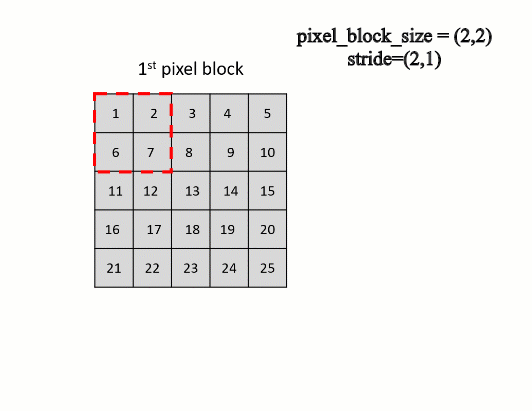# PixelBlockCollection

## Debate

The PixelBlockCollection object is an iterator of all PixelBlock objects in a raster or a list of rasters. It can be used to perform customized raster processing on a block-by-block basis, when otherwise the processed rasters would be too large to load into memory.

The PixelBlockCollection class supports a single raster or a list of rasters as input. Given a single-raster input, the output will have two dimensions, (number of pixel blocks along y, number of pixel blocks along x). For example, to access the first pixel block in the input raster, use the following:

``````pixelblockcoll = PixelBlockCollection(in_raster)
numpy_array = pixelblockcoll.getData()``````

Given a list of rasters as input, the output will have three dimensions, (number of pixel blocks along y, number of pixel blocks along x, number of input rasters). For example, to access the first pixel block from the second raster in the list, use the following:

``````pixelblockcoll = PixelBlockCollection(in_raster1, in_raster2)
numpy_array = pixelblockcoll.getData()``````

The PixelBlockCollection class's stride argument designates the number of bytes from one row of pixels in memory to the next row of pixels in memory. This is also known as increment, pitch, or step size. The following graphic shows how the PixelBlockCollection object iterates through pixels when the stride is set to the default, which is the same size as the pixel block size:The following graphic shows how the PixelBlockCollection object iterates through pixels when the stride is set to (2, 1):## Sintaxis

```PixelBlockCollection
(in_rasters, pixel_block_size, stride, overlay_type, nodata_to_values)```
 Parámetro Explicación Tipo de datos in_rasters[in_rasters,...] The input raster object or a list of raster objects. If you're using a list of raster objects, the rasters must all have the same cell size and spatial reference. Raster pixel_block_size A tuple with two values describing the block size (number of rows, number of columns).(El valor predeterminado es (512, 512)) tuple stride A tuple with two integers specifying the stride or increment along the rows and columns for processing.If no value is specified, the pixel block size will be used, and adjacent pixel blocks will have no overlap.(El valor predeterminado es None) tuple overlay_type Specifies the overlay type when input rasters have different extents. INTERSECTION —Pixel blocks will only cover the area of intersection between rasters.UNION —Pixel blocks will cover the extent of all rasters.(El valor predeterminado es INTERSECTION) String nodata_to_values[nodata_to_values,...] The value to assign to NoData values from the input rasters in the resulting NumPy array. This can be a single value or a list of values, one for each raster. Integer

 Propiedad Explicación Tipo de datos size(Sólo lectura) The number of pixel blocks along the y- and x-directions in the pixel block collection. The number of pixel blocks in the y-direction is in the first position. tuple

## Descripción general del método

 Método Explicación reset () Resets the pixel block collection iterator to the beginning of the collection. shuffle () Shuffles the pixel blocks in the pixel block collection. This is useful when feeding image samples into a deep learning model.

## Métodos

`reset ()`
Valor de retorno
 Tipo de datos Explicación PixelBlockCollection The PixelBlockCollection object that has been reset.
`shuffle ()`
Valor de retorno
 Tipo de datos Explicación PixelBlockCollection The PixelBlockCollection object with pixel blocks in shuffled order.

## Muestra de código

PixelBlockCollection example 1 (Python window)

Iterates through pixel blocks to calculate the total urban area in a land cover raster.

``````import arcpy

# Specify the input raster
in_raster1 = arcpy.Raster("landcover.tif")

# Create a PixelBlockCollection
blockCollection = arcpy.ia.PixelBlockCollection(
in_raster1, pixel_block_size = (512, 512), nodata_to_values = -1)

# Check the number of pixelblocks along x and y direction
number_blocks_y, number_blocks_x= pixelblocks.size

urban_cell_count=0

# Iterate through each PixelBlock
for i in range(number_blocks_y):
for j in range(number_blocks_x):
pixelblock = blockCollection[i][j]
np_array = pixelblock.getData()
urban_cell_count+= np.count_nonzero(np_array == 3)
# value = 3 is urban class

urban_area = urban_cell_count * in_raster1.meanCellWidth*in_raster1.meanCellHeight
print("Total urban area : " + str(urban_area))``````
PixelBlockCollection example 2 (stand-alone script)

Iterates through pixel blocks to calculate the total area that changed from forest in 2006 to urban in 2016.

``````import arcpy
from arcpy.ia import *

# Specify the input rasters
in_raster1 = arcpy.Raster("C:/iapyexamples/data/landcover_2006.tif")
in_raster2 = arcpy.Raster("C:/iapyexamples/data/landcover_2016.tif")

# Create a PixelBlockCollection
blockCollection = arcpy.ia.PixelBlockCollection(
[in_raster1, in_raster2], pixel_block_size = (256, 256), nodata_to_values = -1)

# Check the number of pixelblocks along x and y direction
number_blocks_y, number_blocks_x= pixelblocks.size

forest_to_urban_cell_count = 0

# Iterate through each PixelBlock

for i in range(number_blocks_y):
for j in range(number_blocks_x):
pixelblocklist = blockCollection[i][j]
# get the array from pixelblock in the 1st raster
array_in_raster1 = pixelblocklist.getData()
# get the array from pixelblock in the 2nd raster
array_ in_raster2 = pixelblocklist.getData()

forest_in_raster1= array_in_raster1[array_in_raster1==1] # value = 1 is forest class
urban_in_raster2= array_in_raster2[array_in_raster2==3] # value = 3 is urban class
res= forest_in_raster1+ urban_in_raster2
forest_to_urban_cell_count+= np.count_nonzero(res == 4) # value = 4 is forest in in_raster1 and urban in in_raster2

forest_to_urban_area= forest_to_urban_cell_count * in_raster1.meanCellWidth*in_raster1.meanCellHeight
print("total area from forest in 2006 to urban in 2016 : " + str(forest_to_urban_area))``````